Next: Prompt Selection Up: Implementation Previous: Model Training

## Topic Classification

After the training data is collected and the class models are built, we run the system receiving the audio input from participants of the conversation. The audio input is processed by the speech recognition module which outputs a list of the word candidates as a discounted conversation model. The matching algorithm sequentially updates the conversation model in such a way that the words spoken most recently have the largest score, which is slowly decaying. For a conversation model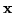, we compute the updates at each step after receiving a word wordk: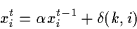(2)

where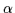is the decay parameter,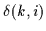equals 1 if the the wordk is the same word as xi (i.e. i = k).

Having computed the conversation model for the time step t, its class-conditional probability is computed as follows: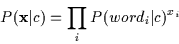(3)

This probability is converted to the posterior probability of the topic c using the Bayes rule. Here, the prior probabilities P(c) of each class are estimated by cross-validation: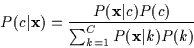(4)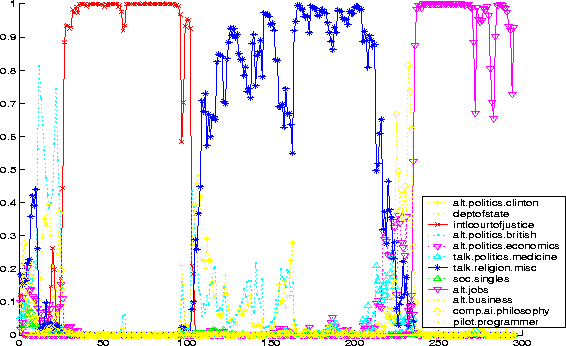Figure 1 shows class probabilities for the ongoing conversation. After these probabilities are computed for each class the most likely topic c is selected and the corresponding feedback is given to the users as described below.Next: Prompt Selection Up: Implementation Previous: Model Training
Tony Jebara
2000-02-24